# Hysteresis curve extraction tool

For Chinese Version: Here; 中文: 这里

This tool uses to extract hysteresis curve from images. Though there may be some software  can get data from image, they need to draw the line in the image, then they get the data from the drawing lines. This tool “Hysteration” could extract data automatically, what it need is the coordinates of three points.

This tool is published in two types: exe and pyc. exe file could be executed in Windows(x64) system. But pyc file could run in Windows, MacOS and Linux system. pyc file is an executable file with python interpreter(v3.5), but it need another two packages: Numpy and OpenCV.

### Tutorial

Before use Hysteration to extract the data from hysteresis curve, we should deal with the origin hysteresis curve image. Here we use Photoshop to simple the image to a black-red image. For a formal readable image, the black curves are the hysteresis curve and the red lines are the coordinate axis. Here are simple rules to form this image: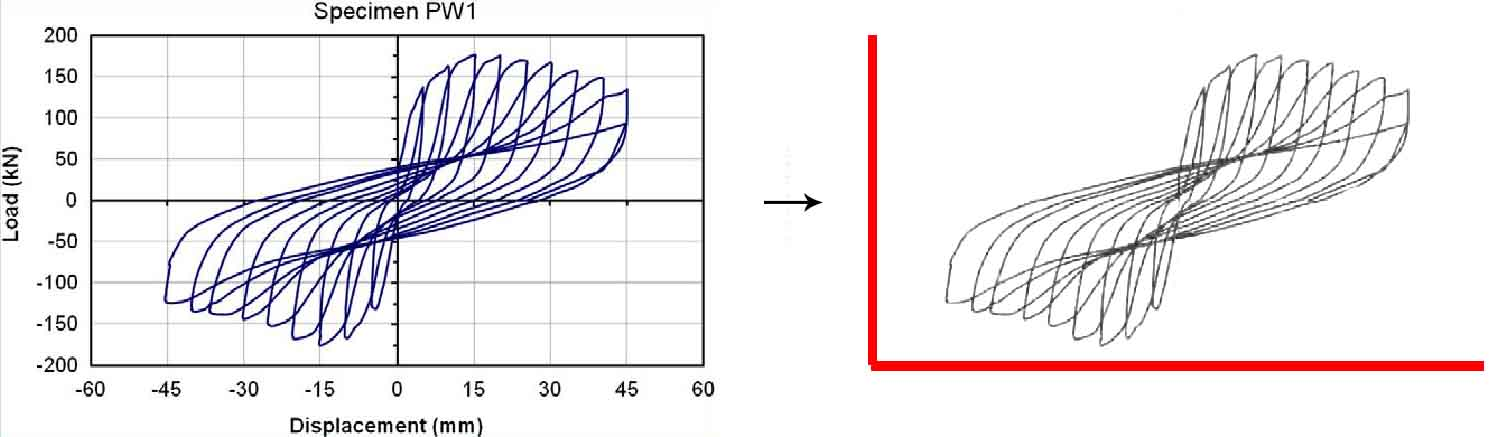Hysteresis curve extraction tool

1. Using red lines to mark the coordinate axis;
2. Turning the hysteresis curve to black curves, keeping the gray scale beyond 127 if the curves are gray;
3. Saving this image to Img.png

Put the Img.png and Hysteration in the same folder, then open Hysteration. I use the following image as an example: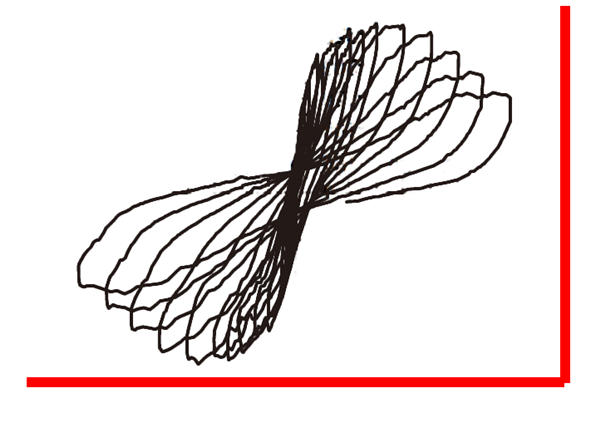Hysteresis curve extraction tool

Keep the format of the image is png, and the name of the image is Img: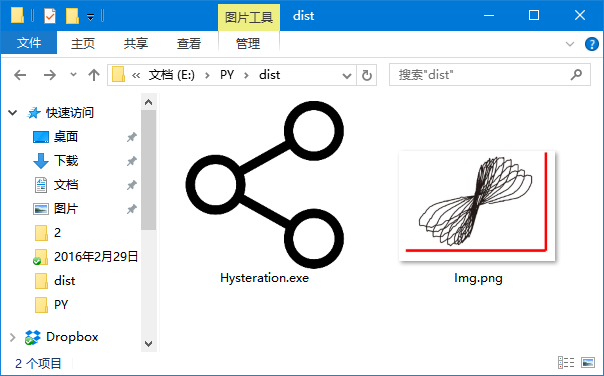Hysteresis curve extraction tool

Open Hysteration and input the origin of the red axis (the coordinate of intersection point of the red lines), the X axis endpoint (the point which is farthest from the origin of the axis in X axis), the Y axis endpoint (the point which is farthest from the origin of the axis in Y axis), using space to split the coordinate.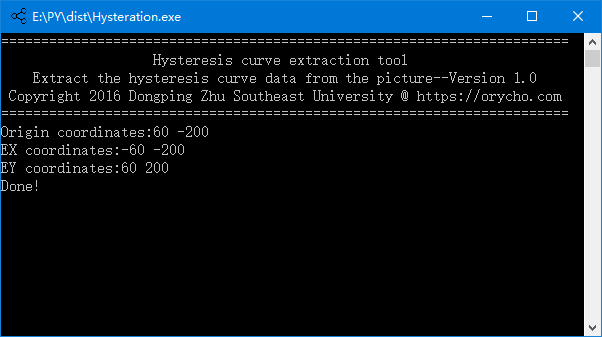Hysteresis curve extraction tool

You will get an Img.csv after extracting the data, csv file contains the points which form the hysteresis curve. Open the Img.csv with MS EXCEL and select scatter plot: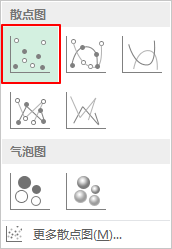Hysteresis curve extraction tool

The following image shows the scatter plot drawing the perfect hysteresis curve from Img.csv: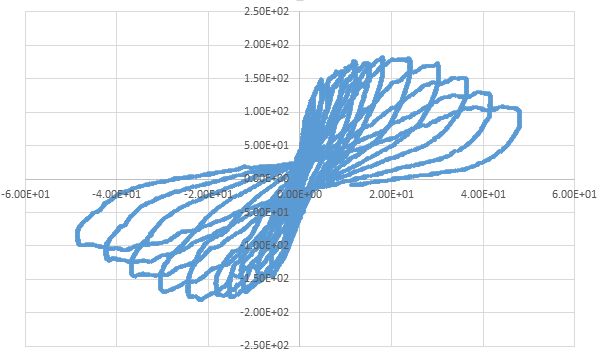Hysteresis curve extraction tool

Hysteration supports different types of coordinate axes, you just need to change the input sequence of the coordinate: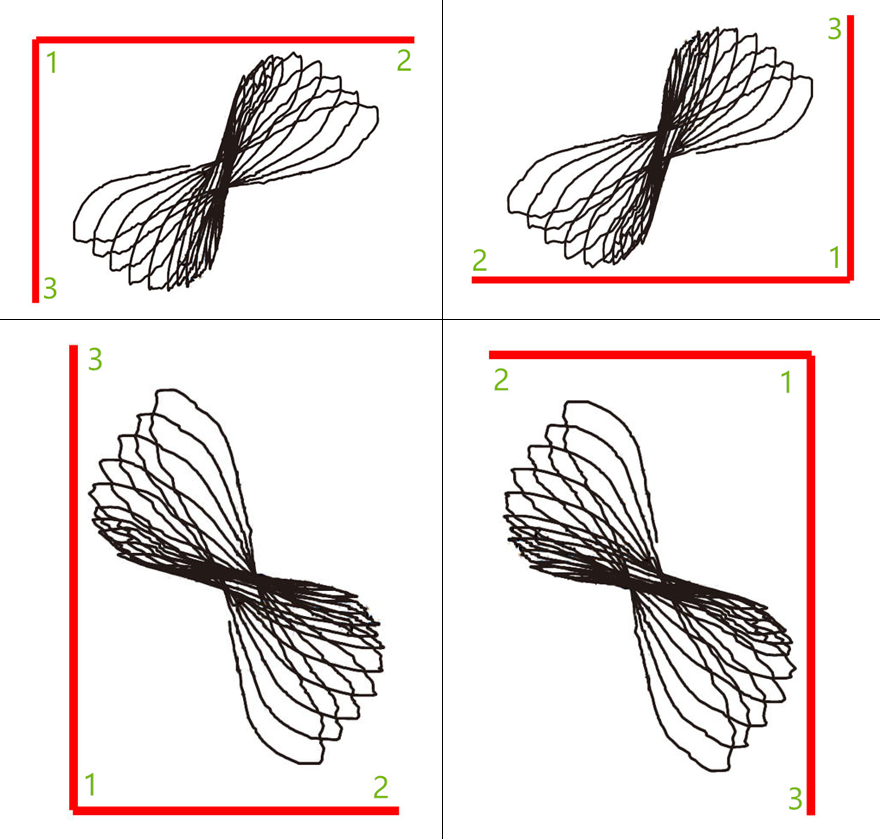Hysteresis curve extraction tool

If the red lines cut cross each other, please remember the point 2 and point 3 in the axis are the points farthest from the point 1.